# Fraction calculator

The calculator performs basic and advanced operations with fractions, expressions with fractions combined with integers, decimals, and mixed numbers. It also shows detailed step-by-step information about the fraction calculation procedure. Solve problems with two, three, or more fractions and numbers in one expression.

## Result:

### 1/2 + 2/5 = 9/10 = 0.9

Spelled result in words is nine tenths.

### How do you solve fractions step by step?

1. Add: 1/2 + 2/5 = 1 · 5/2 · 5 + 2 · 2/5 · 2 = 5/10 + 4/10 = 5 + 4/10 = 9/10
For adding, subtracting, and comparing fractions, it is suitable to adjust both fractions to a common (equal, identical) denominator. The common denominator you can calculate as the least common multiple of both denominators - LCM(2, 5) = 10. In practice, it is enough to find the common denominator (not necessarily the lowest) by multiplying the denominators: 2 × 5 = 10. In the following intermediate step, the fraction result cannot be further simplified by canceling.
In other words - one half plus two fifths = nine tenths.

#### Rules for expressions with fractions:

Fractions - use the slash “/” between the numerator and denominator, i.e., for five-hundredths, enter 5/100. If you are using mixed numbers, be sure to leave a single space between the whole and fraction part.
The slash separates the numerator (number above a fraction line) and denominator (number below).

Mixed numerals (mixed fractions or mixed numbers) write as non-zero integer separated by one space and fraction i.e., 1 2/3 (having the same sign). An example of a negative mixed fraction: -5 1/2.
Because slash is both signs for fraction line and division, we recommended use colon (:) as the operator of division fractions i.e., 1/2 : 3.

Decimals (decimal numbers) enter with a decimal point . and they are automatically converted to fractions - i.e. 1.45.

The colon : and slash / is the symbol of division. Can be used to divide mixed numbers 1 2/3 : 4 3/8 or can be used for write complex fractions i.e. 1/2 : 1/3.
An asterisk * or × is the symbol for multiplication.
Plus + is addition, minus sign - is subtraction and ()[] is mathematical parentheses.
The exponentiation/power symbol is ^ - for example: (7/8-4/5)^2 = (7/8-4/5)2

#### Examples:

subtracting fractions: 2/3 - 1/2
multiplying fractions: 7/8 * 3/9
dividing Fractions: 1/2 : 3/4
exponentiation of fraction: 3/5^3
fractional exponents: 16 ^ 1/2
adding fractions and mixed numbers: 8/5 + 6 2/7
dividing integer and fraction: 5 ÷ 1/2
complex fractions: 5/8 : 2 2/3
decimal to fraction: 0.625
Fraction to Decimal: 1/4
Fraction to Percent: 1/8 %
comparing fractions: 1/4 2/3
multiplying a fraction by a whole number: 6 * 3/4
square root of a fraction: sqrt(1/16)
reducing or simplifying the fraction (simplification) - dividing the numerator and denominator of a fraction by the same non-zero number - equivalent fraction: 4/22
expression with brackets: 1/3 * (1/2 - 3 3/8)
compound fraction: 3/4 of 5/7
fractions multiple: 2/3 of 3/5
divide to find the quotient: 3/5 ÷ 2/3

The calculator follows well-known rules for order of operations. The most common mnemonics for remembering this order of operations are:
PEMDAS - Parentheses, Exponents, Multiplication, Division, Addition, Subtraction.
BEDMAS - Brackets, Exponents, Division, Multiplication, Addition, Subtraction
BODMAS - Brackets, Of or Order, Division, Multiplication, Addition, Subtraction.
GEMDAS - Grouping Symbols - brackets (){}, Exponents, Multiplication, Division, Addition, Subtraction.
Be careful, always do multiplication and division before addition and subtraction. Some operators (+ and -) and (* and /) has the same priority and then must evaluate from left to right.

## Fractions in word problems:

• CupcakesIn a bowl was some cupcakes. Janka ate one third and Danka ate one quarter of cupcakes. a) How many of cookies ate together? b) How many cookies remain in a bowl? Write the results as a decimal number and in notepad also as a fraction.Max is working out 2/3+7/9. He says the answer is 9/12. What mistake have Max made?7 is added to the sum of 4/5 and 6/7
• Samuel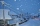Samuel has 1/3 of a bag of rice and Isabella has a 1/2 bag of rice. What fraction of are bag of rice do they have altogether?
• Sum three fractionsWork out the sum of 1/4, 1/5 and 3/10.
• Team runThe first team member in a 926-person relay race must run 2 1/4 laps, the second team member must run 1 1/2 laps, and the third team member must run 3 1/4 laps. How many laps in all must each team run?
• FrankFrank will be riding his bike to school this year. The distance from his house to the end of the street is ⅜ mile. The distance from the end of the street to the school is ⅚ mile. How far is Frank's house from school?
• Jo walks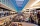Jo walks 3/4 of km to a friends home, 1/2 km to mall, and 2/3 km home. What total distance that joy covers?
• How many 3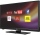How many hours the Andersons watched TV in all Wednesday 3/1 hr Thursdays 2/3 hr Friday 4/5 hr Saturday 3/4 hr2 and 1/8th plus 1 and 1/3rd =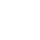Jade was baking cupcakes for her class. She has 4 5/4 cups of flour.  For one batch, she used 1 2/3 cups of flour.  On another batch, she used 7/9 of a cup of flour.  How much flour does Jade have left after making the two batches of cupcakes?Liza, a store owner, buys 560 notebooks. He sold 3/8 of the notebook, and then she adds the stock of a notebook of 1/4 of the number of notebooks she bought. What is the total number of notebooks she purchased?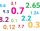Simplify and express the result as a rational number in its simplest form 1/2+ 1/5+ 6.25+0.25